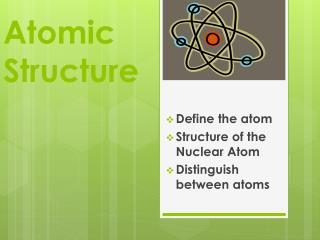Download PresentationAtomic Structure

Atomic Structure - PowerPoint PPT Presentation

Atomic Structure. Define the atom Structure of the Nuclear Atom Distinguish between atoms. In groups of 7 you will research the following scientists’ ideas and contributions to the atomic model.I am the owner, or an agent authorized to act on behalf of the owner, of the copyrighted work described.
Download PresentationAtomic Structure

Download Policy: Content on the Website is provided to you AS IS for your information and personal use and may not be sold / licensed / shared on other websites without getting consent from its author.While downloading, if for some reason you are not able to download a presentation, the publisher may have deleted the file from their server.

- - - - - - - - - - - - - - - - - - - - - - - - - - E N D - - - - - - - - - - - - - - - - - - - - - - - - - -
Presentation Transcript
1. AtomicStructure Define the atom Structure of the Nuclear Atom Distinguish between atoms

2. In groups of 7 you will research the following scientists’ ideas and contributions to the atomic model. • 1. John Dalton, 2. Democritus, 3. J.J Thomson, 4. Ernest Rutherford, 5. Robert Millikan, 6. Eugen Goldstein, 7. James Chadwick • You will present your findings to the class Early Models of the Atom

3. ? Charge ? Location ? Symbol protons What are the 3 kinds of subatomic particles? neutrons electrons

4. 23 V 50.941 Atomic number symbol • The Number of protons (atomic number) • Atoms are electrically neutral; therefore the number of protons (+) must equal the number of electrons (-) Average Atomic mass Try these! What makes one element differ from another? 6

5. Find the difference between the mass number and atomic number Atomic number ? # of protons 14 Si 28.086 # of electrons Average atomic mass ? Round this for mass number How do you find the # of neutrons? Mass number = Atomic number = Number of neutrons=

6. 1.Write the symbol, place the atomic number as the subscript and the mass number as the superscript to the left of the symbol. 14 Si 28.086 Si 28 14 • Also can be written with the name of the symbol • and the mass number Shorthand notation Silicon-28

7. A. • How many neutrons are in each atom? 16 8 O 32 16 S 108 47 Ag practice p+ = e- = n0= p+ = e- = no = p+ = e- = no =

8. Tellurium Remember!!Atomic # = protons = electronsMass # = Protons + NeutronsNeutrons = Mass # - ProtonsProtons = Mass # - Neutrons

9. Atoms that have the same number of protons, but different numbers of neutrons, therefore having different mass numbers • Isotopes are chemically alike due to the # of protons and electrons • There are 3 known isotopes of hydrogen. • Each isotope has one proton • Most common = hydrogen-1 or H • 2nd isotope has one neutron (mass # of 2) = _____________or hydrogen-2 or H • 3rd isotope has 2 neutrons (mass # of 3) = • ___________or hydrogen-3 or H 1 1 2 1 Isotopes 3 1

10. Three isotopes of oxygen are oxygen-16, oxygen-17, oxygen-18. Write the symbol for each, including the atomic number and mass number. • Three isotopes of chromium are chromium-50, chromium-52, and chromium-53. How many neutrons are in each isotope, given that chromium has an atomic number of 24? 16 8 O 17 8 O 18 8 O Practice Chromium-50 = ____ neutrons Chromium-52 = ____neutrons Chromium-53 = ____ neutrons

11. Research common isotopes (radioistopes) • Create a display over commonly used isotopes. • Include the isotope and its use • Also may include pictures, etc. Extra Credit

12. Atomic Mass • Atomic mass unit- (amu)- • Atomic mass= Calculating the atomic mass Multiply the mass of each isotope by the natural abundance, expressed as a decimal, and then add the products

13. Element X has 2 natural isotopes. The isotope with a mass of 10.012 amu (10X) has a relative abundance of 19.91%. The isotope with a mass of 11.009 amu (11X) has a relative abundance of 80.09%. Calculate the atomic mass of this element knowns unknowns Practice Calculate For 10X: 10.012 amu x 0.01991= 1.993 amu For 11X: 11.009 amu x 0.8009 = 8.817 amu For element X: atomic mass =10.810 amu * Atomic mass of element X ? *isotope 10X mass = 10.012 amu rel. abun= 19.91% or 0.1991 *Isotope 11X: mass = 11.009 amu rel. abun.= 80.09% or 0.8009

14. Practice 1. The element of copper has naturally occurring isotopes with mass number of 63and 65. The relative abundance and atomic masses are 69.2% for mass = 62.93 amu, and 30.8% for mass = 64.93 amu. Calculate the average atomic mass of copper 2. Calculate the atomic mass of bromine. The 2 isotopes of bromine have atomic masses and relative abundance of 78.92 amu (50.69%) and 80.92 amu (49.31%)

15. periods Groups 1 2 3 4 5 6 7 8 9 10 11 12 13 14 15 16 17 18 1 2 3 4 5 6 7